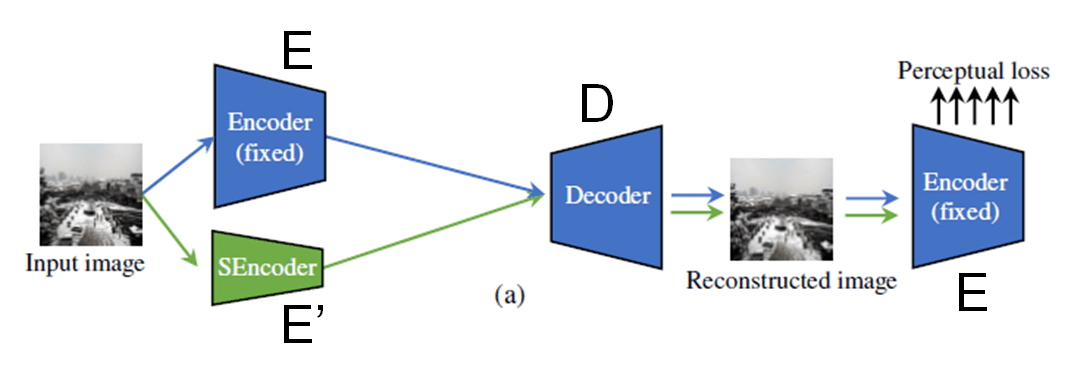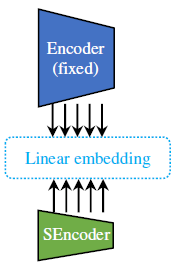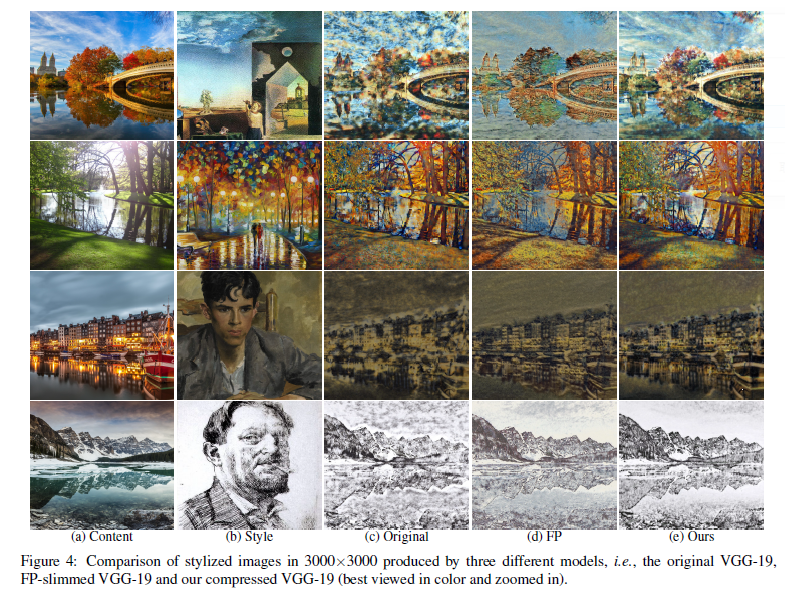# Source

Authors: Huan Wang, Yijun Li, Yuehai Wang, Haoji Hu, Ming-Hsuan Yang
Paper: [CVPR2020] https://arxiv.org/abs/2003.08436
Code: https://github.com/mingsun-tse/collaborative-distillation

# Contributions

1. It proposes a new knowledge distillation method “Collobrative Distillation” based on the exclusive collaborative relation between the encoder and its decoder.
2. It proposes to restrict the students to learn linear embedding of the teacher’s outputs, which boosts its learning.
3. Experimetenal works are done with different stylization frameworks, like WCT and AdaIN.

# Related Works

## Style Transfer

WCT: Li, Y., Fang, C., Yang, J., Wang, Z., Lu, X., & Yang, M. H. (2017). Universal style transfer via feature transforms. arXiv preprint arXiv:1705.08086.
AdaIN: Huang, X., & Belongie, S. (2017). Arbitrary style transfer in real-time with adaptive instance normalization. In Proceedings of the IEEE International Conference on Computer Vision (pp. 1501-1510).

## Model Compression

• low-rank decomposition
• pruning
• quantization
• knowledge distillation
Knowledge distillation is a promising model compression method by transferring the knowledge of large networks (called teacher) to small networks (called student), where the knowledge can be softened probability (which can reflect the inherent class similarity structure known as dark knowledge) or sample relations (which can reflect the similarity structure among different samples).
However, this extra information is mainly label-dependent, thus hardly applicable to low-level tasks. What is the dark knowledge in low-level vision tasks (e.g., neural style transfer) remains an open question.
• compact architecture redesign or search

# Proposed Method

Highlights:
Presumably and confirmed empirically, the decoder D can only work with its matching encoder E like a nut with its bolt.
Notably, they together construct an exclusive collaborative relationship in the stylization process. Since the decoderD is trained to exclusively work with the encoder E, if another encoder E′ can also work with D, it means E′ can functionally play the role of E.Blue arrows show the forward path when training the collaborator network (namely, the decoder). Green arrows show the forward path when the small encoder (“SEncoder”) is trained to functionally replace the original encoder (“Encoder”).

## Training Step 1

To use WCT as an example, for the first step, based on the task at hand, it trains a
collaborator network, i.e., decoder $D$ for the large encoder $E$.

• The encoder E is the commonly choose VGG-19 as the encoder considering its massive capacity and hierarchical architecture. It does not need to train the encoder as the encoder is generalizable.
• Only the decoder is training in the trainging step 1.

The training step 1 is exactly the same as the WCT, with the same loss:

$$\mathcal{L}_{r}^{(k)}=\left\|\mathcal{I}_{r}-\mathcal{I}_{o}\right\|_{2}^{2}+\lambda_{p} \sum_{i=1}^{k}\left\|\mathcal{F}_{q}^{(i)}-\mathcal{F}_{o}^{(i)}\right\|_{2}^{2}$$

where $k \in\{1,2,3,4,5\}$ denotes the kth stage of VGG-19; F(i) denotes the feature maps of the ReLU_i_1 layer; $\lambda_{p}$ is the weight to balance the perceptual loss $\sum_{i=1}^{k}\left\|\mathcal{F}_{q}^{(i)}-\mathcal{F}_{o}^{(i)}\right\|_{2}^{2}$ and pixel reconstruction loss $\left\|\mathcal{I}_{r}-\mathcal{I}_{o}\right\|_{2}^{2}$; $\mathcal{I}_o$ and $\mathcal{I}_r$ denote the original image and reconstructed image, respectively.

## Training Step 2

After obtaining the trained decoder $D$, the second step of the algorithm is to replace the original encoder $E$ with a small encoder $E^′$.

Motivation of the Linear embedding
(1) The small encoder $E^′$ does not have many parameters, so it will actually
form an information bottleneck, slowing down the learningof the student. With these branches plugged into the middlelayers of the network, they will infuse more gradients into the student and thus boost its learning, especially for deep
networks that are prone to gradient vanishing.
(2) In neural style transfer, the style of an image is typically described by the features of many middle layers. Therefore, adding more supervision to these layers is necessary to ensure that they do not lose much the style description power for subsequent use in style transfer.$$\mathcal{L}_{\text {embed }}=\left\|\mathcal{F}-Q \cdot \mathcal{F}^{\prime}\right\|_{2}^{2}$$

The transformation matrix $Q$ is learned through a fully-connected layer without non-linear activation function to realize the linearity assumption. $F$ and $F^′$ are the feature maps of the original encoder $E$ and small encoder $E^′$.

# Experimental Results

Since there are few model compression methods specifically designed for low-level image synthesis tasks, it makes comparison to the filter pruning method. The comparisons are made in the following aspects:

1. Visual Comparison, to see the compressing power of the method.The proposed compressed model tends to produce results with fewer messy textures, while the original model often highlights too many textures in a stylized image. This phenomenon can be explained since a model with fewer parameters has limited capacity, which is less prone to overfitting.

1. User Study
2. Style distance loss
3. Ablation Study for the collaborative distillation and linear embedding losses.
4. Visual comparison to AdaIn and Gatys.
5. Discussion-(1) Filter pruning cannot solve unpleasant messy textures that is not suitable for style transfering.
6. Discussion-(2) Why not use distillation to decoder $D$: When applying distillation to the small decoder, the extra supervision from the original decoder does not help but undermining the effect of style transfering loss,thus deteriorating the visual quality of stylized results.

# Future Direction

The encoder-decoder scheme is also generally utilized in other low-level vision tasks like super-resolution and image inpainting. The performance of the proposed method on these tasks is worth exploring, which the author leaves as the future work.

## Send CommentEdit Comment

|´・ω・)ノ
ヾ(≧∇≦*)ゝ
(☆ω☆)
（╯‵□′）╯︵┴─┴
￣﹃￣
(/ω＼)
∠( ᐛ 」∠)＿
(๑•̀ㅁ•́ฅ)
→_→
୧(๑•̀⌄•́๑)૭
٩(ˊᗜˋ*)و
(ノ°ο°)ノ
(´இ皿இ｀)
⌇●﹏●⌇
(ฅ´ω`ฅ)
(╯°A°)╯︵○○○
φ(￣∇￣o)
ヾ(´･ ･｀｡)ノ"
( ง ᵒ̌皿ᵒ̌)ง⁼³₌₃
(ó﹏ò｡)
Σ(っ °Д °;)っ
( ,,´･ω･)ﾉ"(´っω･｀｡)
╮(╯▽╰)╭
o(*////▽////*)q
＞﹏＜
( ๑´•ω•) "(ㆆᴗㆆ)

Emoji